# What is Dielectric Film Measurement and How Does it Work?

The measured ellipsometric parameters (Ψ and Δ) include the physical properties of the layer structure being analyzed. This information shows the layer thickness and refractive index concerning the fundamental equation of ellipsometry.

As the equation is transcendental and very nonlinear, it is necessary to solve the equation using numerical methods on a model-based perspective. During the method, the layer structure is analyzed in terms of the thickness and optical functions of the model.

The relative phase shift is measured and placed in comparison with measured quantities through a numerical regression process.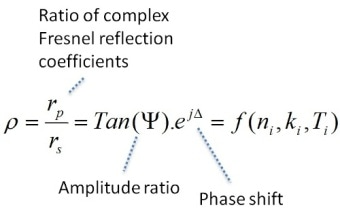## Optical Modeling

When the numerical regression process is taking place, a loose optical model of the structure needs to be deciphered. A substrate is often estimated using a semi-infinite material that is thick and has known optical characteristics.

Parametric functions are often used to outline the spectral absorption and dispersion of each layer. Depending on the material, basic empirical formulas can be utilized or more multifaceted definitions can be used.

Where a parametric function has occurred, for example in the case of the Cauchy law, the dispersion is parametrized by more than a few parameter values. In this case, A, B, and C, are known to be useful parameters when regression has taken place. If the parameters are collected at the end of the regression process, the refractive index can then be measured as a function of wavelength.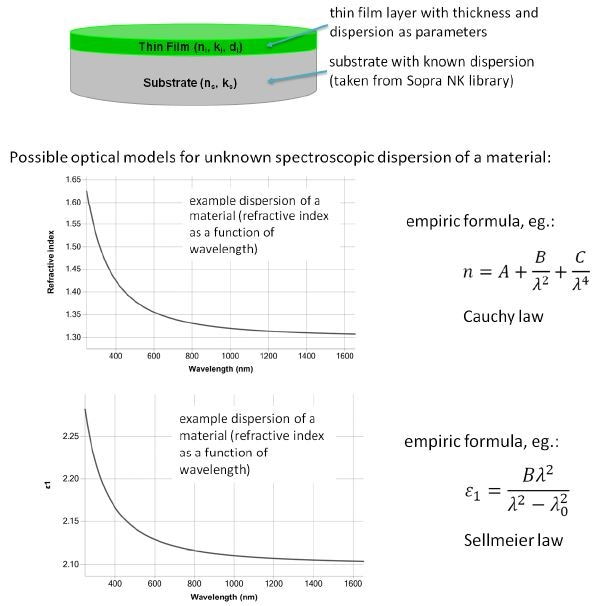Another option for modeling the dielectric function is the Sellmeier model, whereas the Cauchy law is an empirical formula. This model is founded on a zero linewidth Lorentzian absorption peak located away from the range of measurement at peak position λ0 and oscillator strength B. Therefore, the film has no optical absorption within the wavelength range being measured.

Its hyperbolic-shape can be equal to those obtained using the Cauchy model. The benefits of utilizing the Sellmeier model is that it can be more simply combined with alternative dispersion formulas refined for complex dielectric function. For example, Gauss, Drude, or Lorentz. It is an applicable model for wider wavelength range modeling, and can also capture more than one Sellmeier peak in a single model.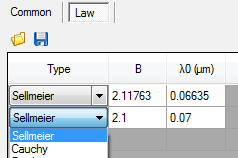SEA software selection of dispersion law types in Structure tab

## Performance of Dielectric Film Measurement

The range of sensitivity for the measurement of optically transparent films can differ from sub-nanometers to several tens of micrometers. The simulation result demonstrates clearly sub-nm sensitivity, for example, for SiO2 covered Si substrate in the Δ curve above 600 nm wavelength.

The higher limit of determining dielectric film thickness is dependant on the maximum wavelength range and resolution created for the spectroscopic ellipsometer instrument used. When glass substrate is used, the refractive index variation between the thin film coating and the substrate is extremely reduced in the context of the Si02 and Si sample structure.

As such, SiO2 or glass measurements need instruments that are of a high sensitivity that can optically distinguish between two dielectrics that are similar. By using Semilab’s rotating compensator ellipsometer, one can measure SiO2 thickness on glass sheets even where the thickness range is as low as <50 nm.

In recent times, this method of application is increasingly relevant for the flat-panel display industry where the oxide coating has the purpose of acting as an initial buffer layer, and must be comparably deposited along the several m2 area panels of glass.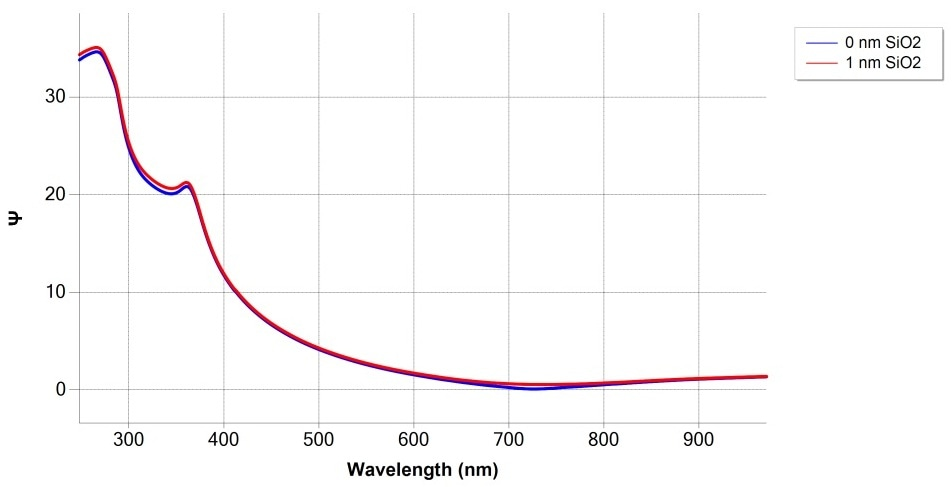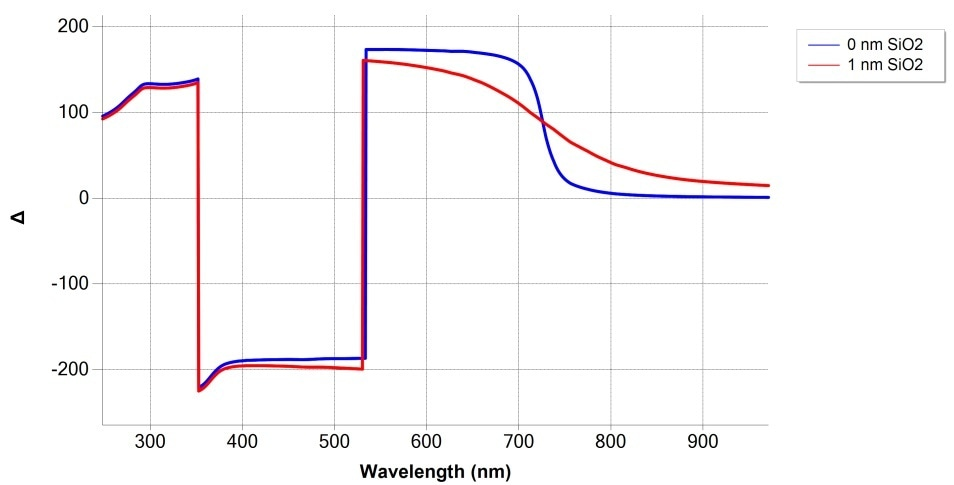Simulation of SiO2/Si measurement at 75° angle of incidence, with and without 1 nm SiO2 film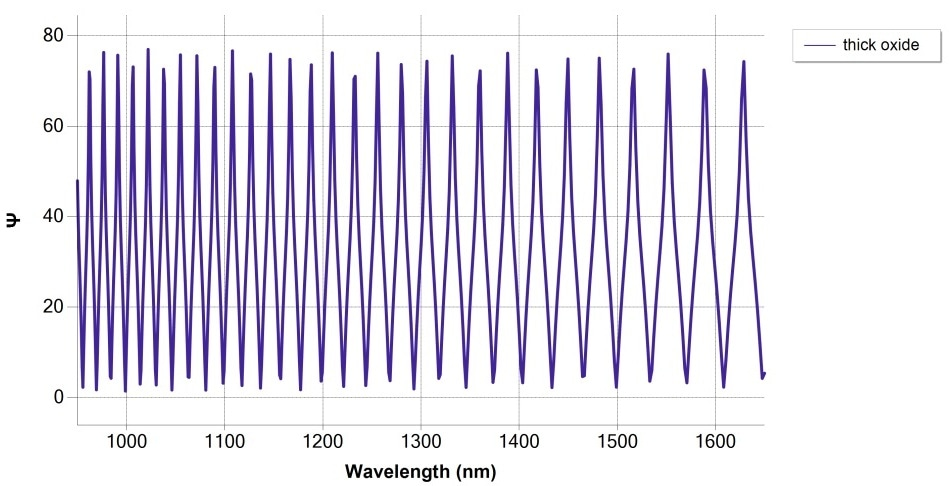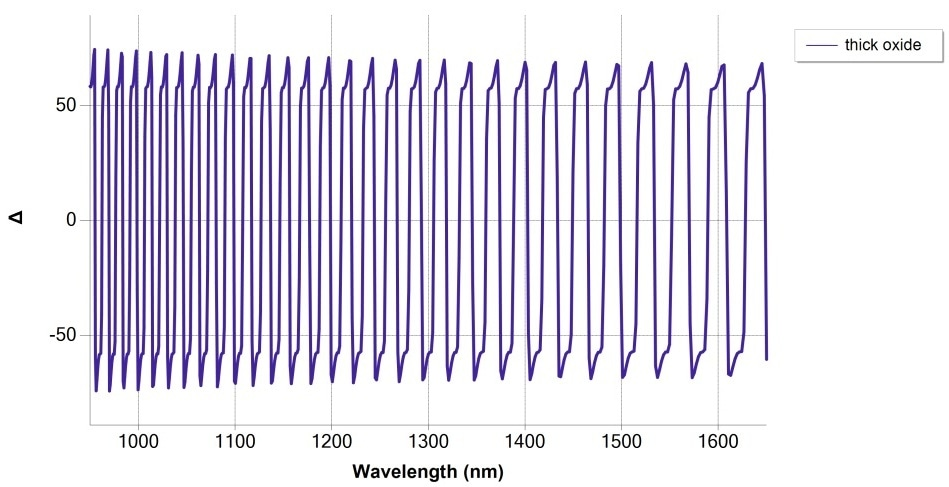Simulation of SiO2/Si measurement at 75° angle of incidence, with thickness 30 μm with NIR fast detection spectrograph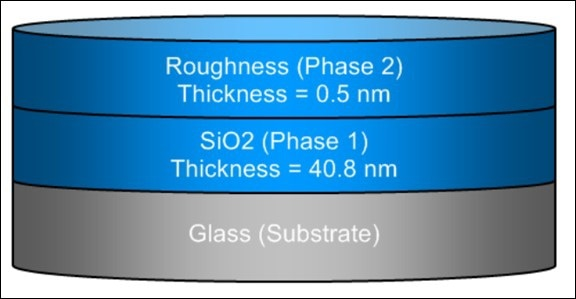Optical model result output for the sample structure of thin SiO2/glass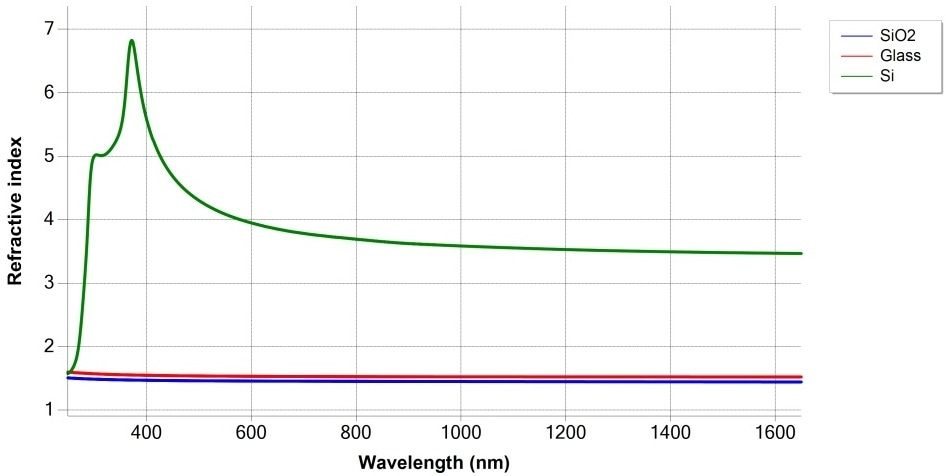Refractive index difference for SiO2 layer vs. glass or Si substrates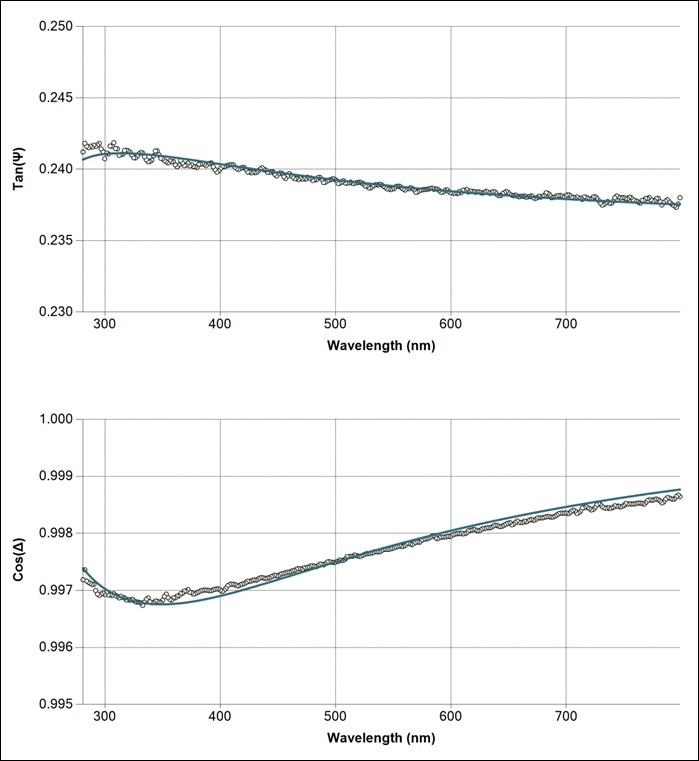Measured and fitted spectrum of thin SiO2/glass sample at 65° angle of incidenceThis information has been sourced, reviewed and adapted from materials provided by Semilab Semiconductor Physics Laboratory.

## Citations

• APA

Semilab Semiconductor Physics Laboratory. (2019, December 24). What is Dielectric Film Measurement and How Does it Work?. AZoM. Retrieved on October 31, 2020 from https://www.azom.com/article.aspx?ArticleID=17800.

• MLA

Semilab Semiconductor Physics Laboratory. "What is Dielectric Film Measurement and How Does it Work?". AZoM. 31 October 2020. <https://www.azom.com/article.aspx?ArticleID=17800>.

• Chicago

Semilab Semiconductor Physics Laboratory. "What is Dielectric Film Measurement and How Does it Work?". AZoM. https://www.azom.com/article.aspx?ArticleID=17800. (accessed October 31, 2020).

• Harvard

Semilab Semiconductor Physics Laboratory. 2019. What is Dielectric Film Measurement and How Does it Work?. AZoM, viewed 31 October 2020, https://www.azom.com/article.aspx?ArticleID=17800.